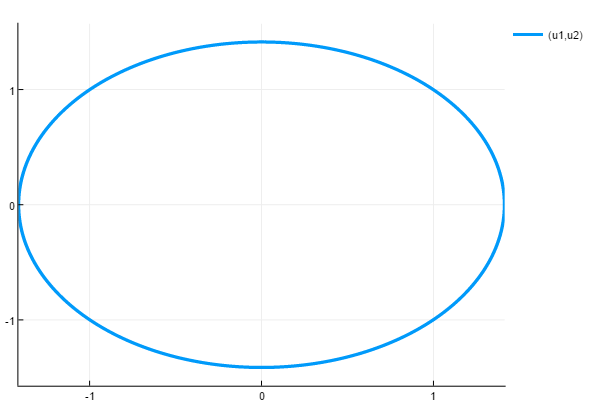## DiffEqCallbacks.jl

A library of useful callbacks for hybrid scientific machine learning (SciML) with augmented differential equation solvers
Popularity
52 Stars
Updated Last
7 Months Ago
Started In
January 2017

# DiffEqCallbacks.jl: Prebuilt Callbacks for extending the solvers of DifferentialEquations.jl

DifferentialEquations.jl has an expressive callback system which allows for customizable transformations of te solver behavior. DiffEqCallbacks.jl is a library of pre-built callbacks which makes it easy to transform the solver into a domain-specific simulation tool.

## Tutorials and Documentation

For information on using the package, see the stable documentation. Use the in-development documentation for the version of the documentation, which contains the unreleased features.

## Manifold Projection Example

Here we solve the harmonic oscillator:

```u0 = ones(2)
function f(du,u,p,t)
du = u
du = -u
end
prob = ODEProblem(f,u0,(0.0,100.0))```

However, this problem is supposed to conserve energy, and thus we define our manifold to conserve the sum of squares:

```function g(resid,u,p,t)
resid = u^2 + u^2 - 2
resid = 0
end```

To build the callback, we just call

`cb = ManifoldProjection(g)`

Using this callback, the Runge-Kutta method `Vern7` conserves energy. Note that the standard saving occurs after the step and before the callback, and thus we set `save_everystep=false` to turn off all standard saving and let the callback save after the projection is applied.

```sol = solve(prob,Vern7(),save_everystep=false,callback=cb)
@test sol[end]^2 + sol[end]^2 ≈ 2```### Required Packages

View all packages

### Used By Packages

View all packages